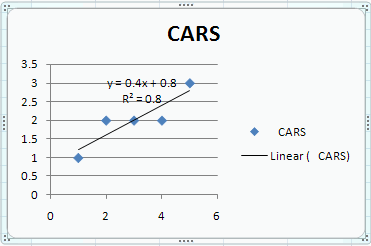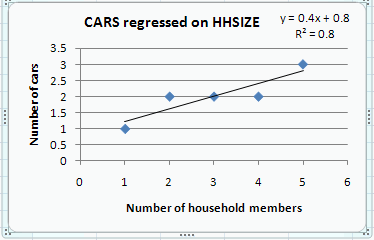## A. Colin Cameron, Dept. of Economics, Univ. of Calif. - Davis

This January 2009 help sheet gives information on
• Two-way scatter plots.
• Trendline (regreesion line) for two-way scatter plots.
• Customizing the two-way scatter plot.
For considerable detail on the basics of chart making see:  Excel 2007: Charts (column, pie, line)

TWO-WAY SCATTERPLOTS

A two-way scatter plot has one variable (say Y) on the vertical axis and one variable (say X) on the horizontal axis.
This is done by choosing the Insert Tab and the Charts Group and Scatter option.

We use data in file carsdata.xls to produce a scatterplot with CARS on the vertical axis (Y-axis) and HHSIZE on the horizontal axis (X-axis).

The scatterplot is an XY scatterplot, so the data in the Excel spreadsheet should be in two adjoining columns, with the X variable followed by the Y variable.
For our example we need HHSIZE followed by CARS.

• So we first copy column A to column C.
• Then highlight columns B and C.
• Then choose the Insert Tab and the Charts Group and Scatter and then Scatter Only with markersThis yields the following plotTRENDLINE FOR TWO-WAY SCATTERPLOTS

A separate trendline (or regression line) can be added after the chart is created by

• Clicking on a point within the chart so that all the point on the chart are highlighted (this is tricky)
• Right-click to get options including Add Trendline
We haveThis yields the Format Trendline dialog box.
• Select linear (the default)
• Select Display Equation on chart
• Select Display R-Squared value on chartHitting Close adds a trendline to the data.CUSTOMIZING THE TWO-WAY SCATTERPLOT

The look of the scatter plot can be improved. We do the following

• Delete the redundant legend on the right (CARS --- Linear (CARS)
• Move the equation formula and R^2  (drag the box).
• Change the heading text and reduce the font
• Add a title for the horizontal access as follows
- In Chart Tools choose Layout then Axis Titles Primary Horizontal Axis Title then Title below Access
- Right in the title in the title box
• Add a title for the horizontal access as follows
- In Chart Tools choose Layout then Axis Titles Primary Vertical Axis Title then Rotated Title below Access
- Right in the title in the title box

We obtainFor further information on how to use Excel go to
http://cameron.econ.ucdavis.edu/excel/excel.html